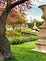# Best of Modern JavaScript — Destructuring and DefaultsPhoto by Todd Quackenbush on Unsplash

Since 2015, JavaScript has improved immensely.

It’s much more pleasant to use it now than ever.

In this article, we’ll look at JavaScript destructuring.

# Array Patterns Work with Iterable Object

We can use array patterns like the rest operator with iterable objects.

For example, we can write:

`const [x, ...y] = 'foo';`

The `x` is `'f'` and `y` is `['o', 'o']` .

Since strings are iterable, we can destructure them.

Destructuring also works with string code points.

For example, we can write:

`const [x, y, z] = 'a\uD83D\uDCA9c';`

The `x` is `'a'` , `b` is `'\uD83D\uDCA9'` and `z` is `'c'` .

# Default Values

We can set default values when destructuring.

For example, we can write:

`const [x = 13, y] = [];`

Then `x` is 13 and `y` is `undefined` since the array is empty.

Since 13 is the default value of `x` , it’s assigned to it.

Therefore `undefined `triggers default values to be assigned.

Default values are computed on demand.

If they aren’t needed, then they won’t be used.

For example, if we have:

`const {  prop: y = fn()} = foo;`

Then that’s the same as:

`let y;if (foo.prop === undefined) {  y = fn();} else {  y = foo.prop;}`

Default values can refer to other variables in the pattern.

For instance, we can write:

`const [x = 13, y = x] = [];`

Then `x` and `y` are both 13.

If we have:

`const [x = 3, y = x] = ;`

Then `x` and `y` are both 17.

And if we write:

`const [x = 3, y = x] = [7, 4];`

Then `x` is 7 and `y` is 4.

The default value is only used when there’s no corresponding value on the right side.

We can also have default object values when destructuring.

For example, we can write:

`const [{  prop: x} = {}] = [];`

Then `x` is an empty object since there’s nothing on the right side.

If we have:

`const {  prop: x} = {};`

we get the same thing.

If we have:

`const [{  prop: x} = {  prop: 'foo'}] = [];`

Then `x` is `foo` since we assigned an object to the left side with the default value.

However, if we have:

`const [{  prop: x} = {  prop: 'foo'}] = [{}];`

Then `x` us `undefined` since we have an object in the array on the right side, so the default value won’t be assigned on the left side.

If we want to trigger the default value assignment, then we’ve to assign a default value to the property itself:

`const [{  prop: x = 'foo'} = {}] = [{}];`

Then `x` would be `'foo'` since `prop` isn’t found on the right side.

# Property Value Shorthands

Property value shorthand works like we expect with destructuring as we can see from the previous examples.

So:

`const {  x,  y} = {  x: 1,  y: 2};`

is the same as:

`const {  x: x,  y: y} = {  x: 1,  y: 2};`Photo by Behzad Ghaffarian on Unsplash

# Conclusion

Destructuring can be done with objects and arrays.

There’re many tricks with default values.

Web developer. Subscribe to my email list now at https://thewebdev.info/subscribe/. Email me at hohanga@gmail.com

Web developer. Subscribe to my email list now at https://thewebdev.info/subscribe/. Email me at hohanga@gmail.com